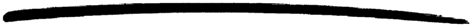### DESCRIPTION

overload is a process which indirectly regulates CPU usage in the system. The idea is that a main application calls the request/0 function before starting a major job, and proceeds with the job if the return value is positive; otherwise the job must not be started.

overload is part of the sasl application, and all configuration parameters are defined there.

A set of two intensities are maintained, the total intensity and the accept intensity. For that purpose there are two configuration parameters, the MaxIntensity and the Weight value (both are measured in 1/second).

Then total and accept intensities are calculated as follows. Assume that the time of the current call to request/0 is T(n), and that the time of the previous call was T(n-1).

• The current total intensity, denoted TI(n), is calculated according to the formula,
TI(n) = exp(-Weight*(T(n) - T(n-1)) * TI(n-1) + Weight,
where TI(n-1) is the previous total intensity.
• The current accept intensity, denoted AI(n), is determined by the formula,
AI(n) = exp(-Weight*(T(n) - T(n-1)) * AI(n-1) + Weight,
where AI(n-1) is the previous accept intensity, provided that the value of exp(-Weight*(T(n) - T(n-1)) * AI(n-1) is less than MaxIntensity; otherwise the value is
AI(n) = exp(-Weight*(T(n) - T(n-1)) * AI(n-1).

The value of configuration parameter Weight controls the speed with which the calculations of intensities will react to changes in the underlying input intensity. The inverted value of Weight,

T = 1/Weight

can be thought of as the "time constant" of the intensity calculation formulas. For example, if Weight = 0.1, then a change in the underlying input intensity will be reflected in the total and accept intensities within approximately 10 seconds.

The overload process defines one alarm, which it sets using alarm_handler:set_alarm(Alarm). Alarm is defined as:

This alarm is set when the current accept intensity exceeds MaxIntensity.

A new overload alarm is not set until the current accept intensity has fallen below MaxIntensity. To prevent the overload process from generating a lot of set/reset alarms, the alarm is not reset until the current accept intensity has fallen below 75% of MaxIntensity, and it is not until then that the alarm can be set again.

### EXPORTS

Returns accept or reject depending on the current value of the accept intensity.

The application calling this function should be processed with the job in question if the return value is accept; otherwise it should not continue with that job.

Types:

OverloadInfo = [{total_intensity, TotalIntensity}, {accept_intensity, AcceptIntensity}, {max_intensity, MaxIntensity}, {weight, Weight}, {total_requests, TotalRequests}, {accepted_requests, AcceptedRequests}].
TotalIntensity = float() > 0
AcceptIntensity = float() > 0
MaxIntensity = float() > 0
Weight = float() > 0
TotalRequests = integer()
AcceptedRequests = integer()

Returns the current total and accept intensities, the configuration parameters, and absolute counts of the total number of requests, and accepted number of requests (since the overload process was started).